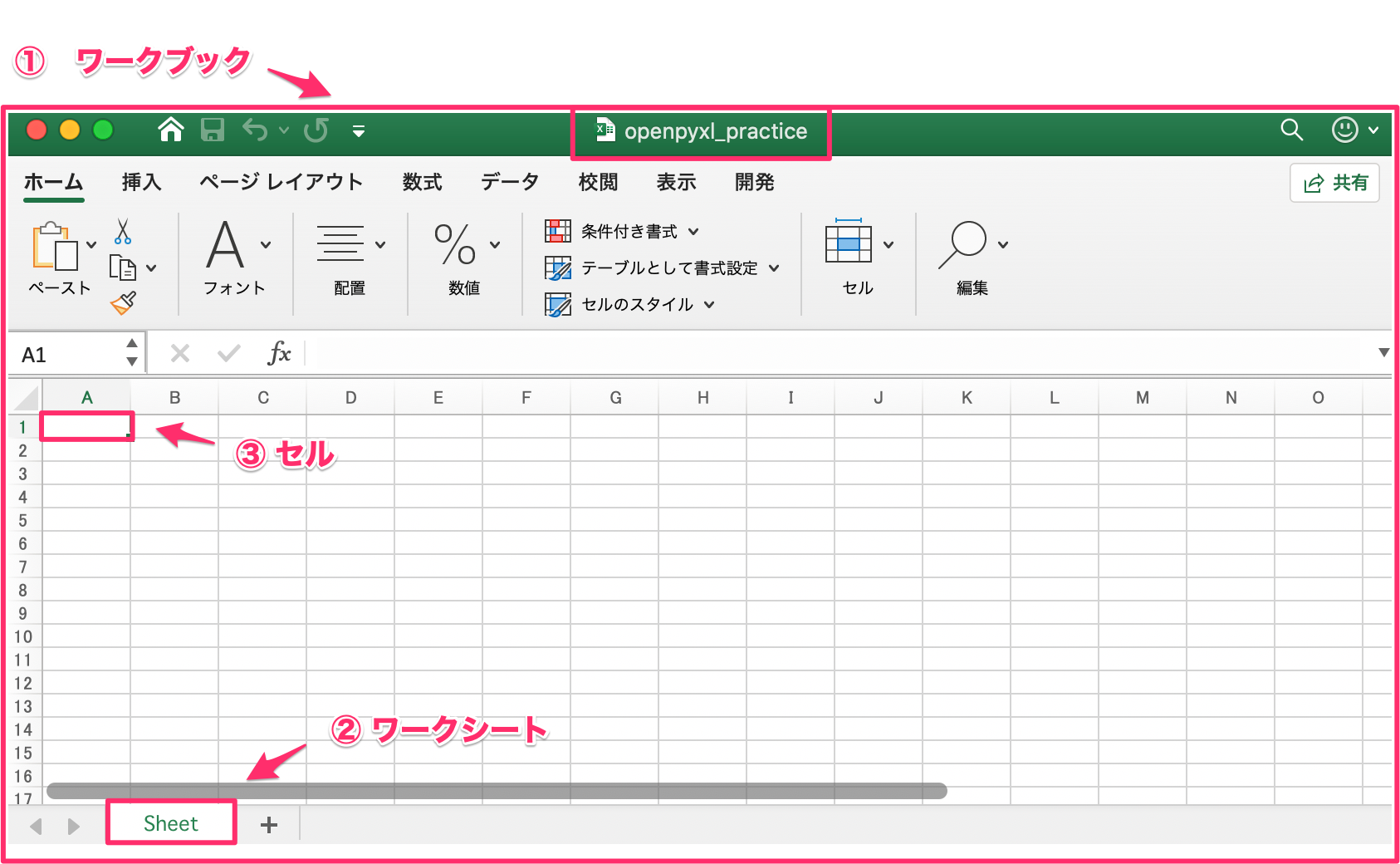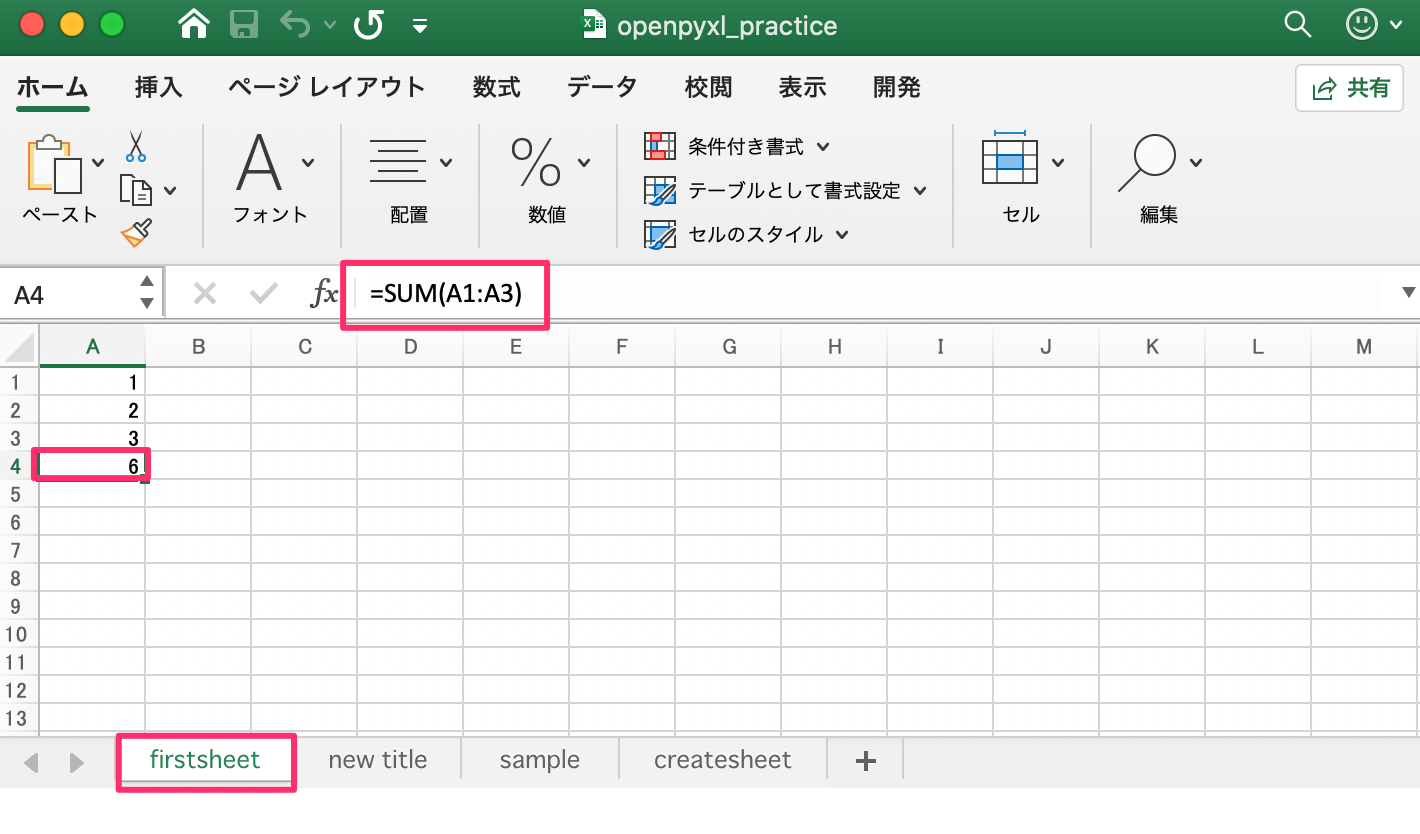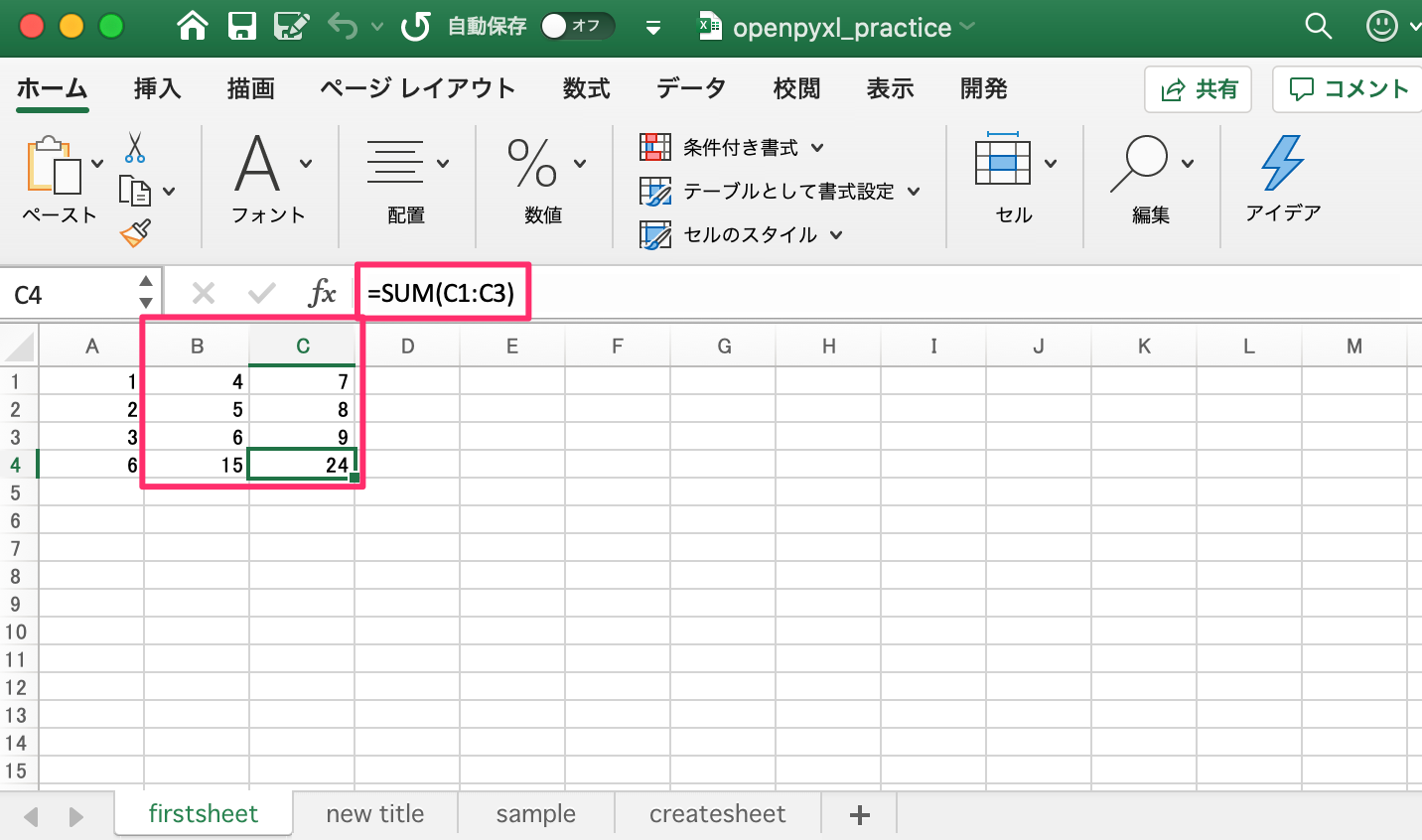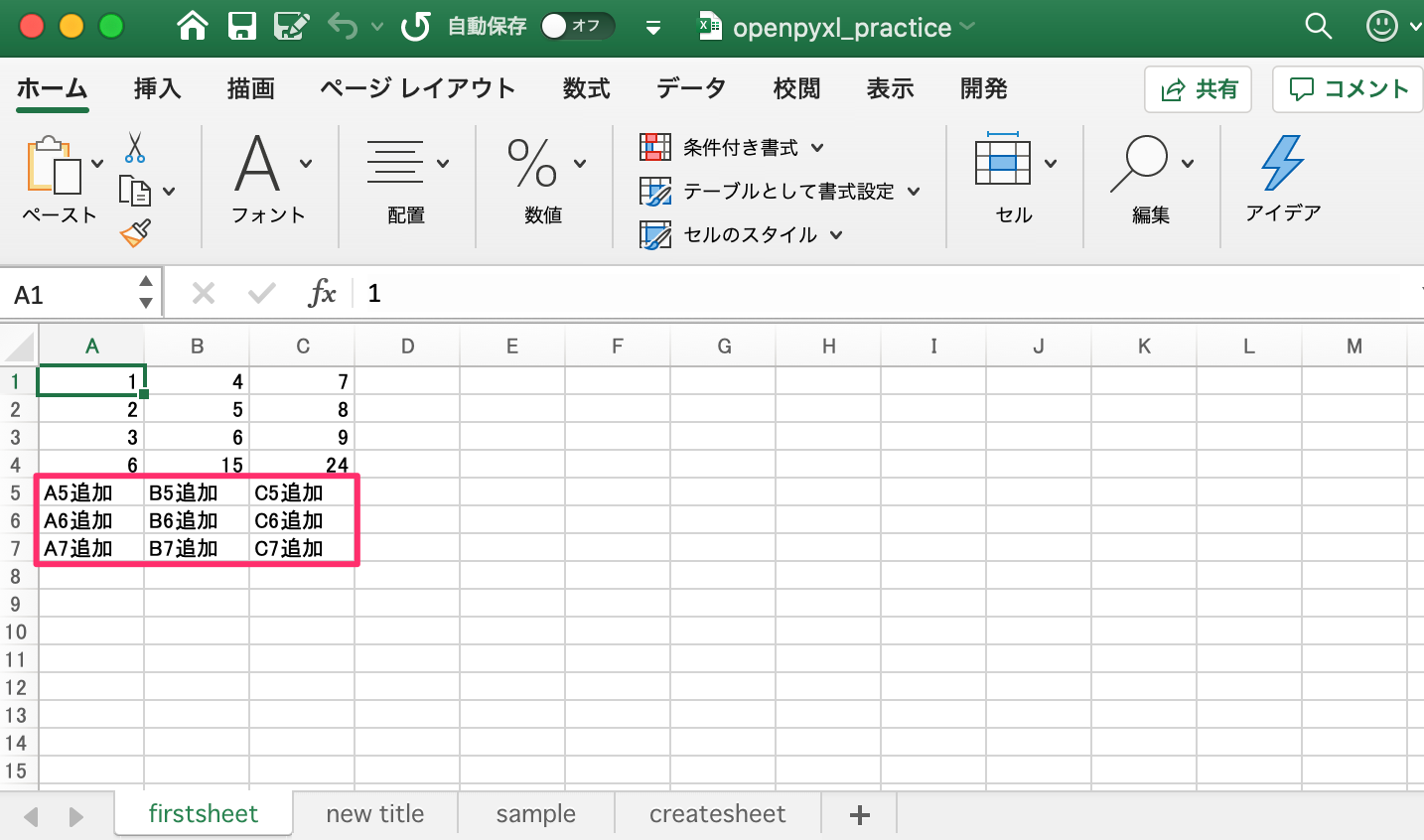# 【OpenPyXL】对 Excel 单元格的操作Tango

OpenPyXL 对 Excel 的操作，大致分三步，把冰箱门打开，把大象放进去....错了，重来：

1. 获取 Excel 文件(workbook)

2. 获取 Sheet 对象(worksheet)

3. 获取单元格(cell)

ワークブック==workbook

ワークシート==worksheet

セル==cell## 单一单元格的操作

1. 一个一个的输入

import openpyxlwb = openpyxl.load_workbook('openpyxl_practice.xlsx')ws = wb.active  # 「firstsheet」  # 对A1~A3输入值ws['A1'] = 1ws['A2'] = 2ws['A3'] = 3ws['A4'] = '=SUM(A1:A3)'  # 也可以直接输入函数# R1C1的形式ws.cell(row = 1, column = 2).value = 4               # B1ws.cell(row = 2, column = 2).value = 5               # B2ws.cell(row = 3, column = 2).value = 6               # B3ws.cell(row = 4, column = 2).value = '=SUM(B1:B3)'   # B4 # 下面这种方式也可以ws.cell(row = 1, column = 3, value = 7)              # C1ws.cell(row = 2, column = 3, value = 8)              # C2ws.cell(row = 3, column = 3, value = 9)              # C3ws.cell(row = 4, column = 3, value = '=SUM(C1:C3)')  # C41. 一个一个的取得

# A1的形式a1 = ws['A1'].value                     # A1的值取得print(a1) # R1C1的形式r1c1 = ws.cell(row=1, column=2).value   # B1的值取得print(r1c1)

## 多个单元格的操作

1. 多个单元格输入

# 同一行中，多个单元格同时输入datas = ["A5追加", "B5追加", "C5追加"]ws.append(datas)  # 复数行中，多个单元格同时输入datas = [          ["A6追加", "B6追加", "C6追加"],          ["A7追加", "B7追加", "C7追加"],        ] for row_data in datas:    ws.append(row_data)1. 按行获取多个单元格数据

row = ws  # 第3行print(row)

# 按照行，获取指定单元格数据print("--- C3的数据 ---")row = ws  # 第3行print(row.value)    # C3（值：9）  # 获取整行说有单元格的数据print("--- 第3行所有的数据 ---")row = ws  # 第3行 for row_data in row:    print(row_data.value)

row = ws  # 第4行 for row_data in row:    print(row_data.value)

import openpyxl # 如果要获取函数运算后的结果，添加「data_only=True」wb = openpyxl.load_workbook('openpyxl_practice.xlsx', data_only=True)ws = wb.active  # 获取有函数的行row = ws  # 第4行 for row_data in row:    print(row_data.value)

# 复数行的数据获取row_range = ws[3:4]  # 第3行和第4行 for row_data in row_range:  # 遍历每一行    for data in row_data:   # 遍历改行的每一列（A3,B3,C3）        print(data.value)

# 复数行的数据获取row_range = ws['A3:B4']  # A3〜B4 for row_data in row_range:  # 第3行    for data in row_data:   # 改行的列（A3,B3）        print(data.coordinate, data.value)

# 获取整列的数据col = ws['C']  # C列 for col_data in col:    print(col_data.value)

# 获取复数列col_range = ws['A:B']  # A列 B列 for col_data in col_range:  #  遍历列    for data in col_data:   #  遍历该列的每一行的数据        print(data.coordinate, data.value)

for row in ws.values:    print(row)

### iter_rows()和 iter_cols()方法

# 获取A1~C2的值print("--- 获取整行数据---")for row in ws.iter_rows(min_row = 1, max_col = 3, max_row = 2, values_only=True):    print(row) print("--- 每个单元格的数据 ---")for row in ws.iter_rows(min_row = 1, max_col = 3, max_row = 2, values_only=True):    for data in row:        print(data)

# A1~B4的值print("--- 获取整列的数据 ---")for col in ws.iter_cols(min_row = 1, max_col = 2, max_row = 4, values_only=True):    print(col) print("--- 每个单元格的数据 ---")for col in ws.iter_cols(min_row = 1, max_col = 2, max_row = 4, values_only=True):    for data in col:        print(data)

ws.max_column:获得最终列

ws.max_row:获得最终行### Tango

【坐标】无锡 【元坐标】黑龙江/北极村 【软件技能】Java，C#，Python 【爱好】炉石传说 【B站】https://space.bilibili.com/397260706/ 【个人站】www.it-worker.club

## 评论 (3 条评论)2021 年 03 月 28 日 12:41

2021 年 03 月 28 日 18:48

2021 年 03 月 29 日 10:54